# Mathematics

Sort By:

MathematicsIn this course, Professor Paul Allen (Lewis & Clark College) explains the main ideas behind calculus, with the hope that we can keep this big picture in mind when learning calculus computation techniques. In the first mini-lecture, we discuss the...

4 lectures

0:43:04

Prof. Paul T. Allen

Lewis & Clark College

Mathematics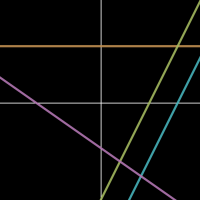In this course, Professor Keith Ball (University of Warwick) gives an introduction to graphs, covering topics A8-A10 in the Pearson Edexcel GCSE (9-1) Mathematics (1MA1) Specification for Foundation Tier. In the first mini-lecture, we provide...

7 lectures

0:50:00

Prof. Keith Ball

Warwick University

MathematicsIn this course, Professor Kevin Buzzard (Imperial College London) explores numbers, in particular the counting numbers and their abstract nature. In the first mini-lecture, we consider what ‘2 + 2 = 4’ really means and introduce the idea of an...

8 lectures

0:55:30

Prof. Kevin Buzzard

Imperial College London

Mathematics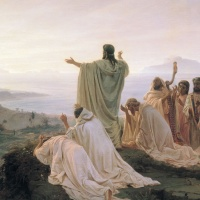In this course, Dr Piers Bursill-Hall (University of Cambridge) debunks the myths of Pythagoras and the Pythagorean theorem. In the first mini-lecture, we learn about the Pythagoreans, a religious cult supposedly following the teachings and...

7 lectures

1:13:58

Dr Piers Bursill-Hall

University of Cambridge

MathematicsIn this course, Professor Keith Ball (University of Warwick) explores solving equations, covering Topics A17-A18 in the Pearson Edexcel GCSE (9-1) Mathematics (1MA1) Specification for Higher Tier. In the first mini-lecture, we give an introduction...

7 lectures

1:16:35

Prof. Keith Ball

Warwick University

MathematicsIn this course, Professor Satyan Devadoss (University of San Diego) explores some of the most fascinating unsolved problems in mathematics. In the first mini-lecture, we discuss reasons why we should care about cutting-edge mathematics,...

5 lectures

0:52:20

University of San Diego

MathematicsIn this course, Professor Colin Adams (Williams College) explores tiling theory. In the first mini-lecture, we think about tilings found in everyday life and give the mathematical definition of a tiling. In the second mini-lecture, we learn how to...

5 lectures

0:52:26

Williams College

Mathematics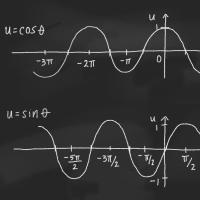In this course, Professor Thomas Garrity (Williams College) explores the big picture of trigonometry — specifically that of sines and cosines — in the modern world of mathematics and in our daily lives. In the first mini-lecture, we define the...

6 lectures

0:58:01

Prof. Thomas Garrity

Williams College

Mathematics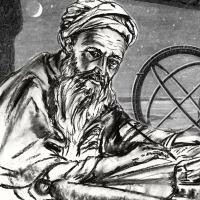In this course, Dr Piers Bursill-Hall (University of Cambridge) explores the origins of algebra: Hindu-Arabic arithmetic. In the first mini-lecture, we consider types of arithmetic used by ancient peoples, including Egyptians, Mesopotamians, and...

7 lectures

1:15:52

Dr Piers Bursill-Hall

University of Cambridge

Mathematics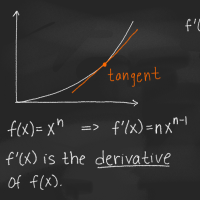In this course, Professor Samir Siksek (University of Warwick) gives an introduction to differentiation for AS and A-Level students in the first of his three-course series on this topic. In the first mini-lecture, we recall the equation of a...

5 lectures

0:40:27

Prof. Samir Siksek

Warwick University

MathematicsIn this course, Professor Keith Ball (University of Warwick) gives an introduction to graphs, covering topics A8-A10 in the Pearson Edexcel GCSE (9-1) Mathematics (1MA1) Specification for Higher Tier. In the first mini-lecture, we provide...

7 lectures

0:58:28

Prof. Keith Ball

Warwick University

Mathematics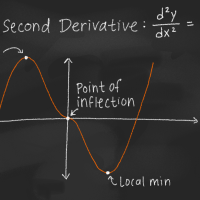In this course, Professor Samir Siksek (University of Warwick) concludes his discussion of differentiation for A-Level students in the third of this three-course series. In the first mini-lecture, we introduce the second derivative and work...

4 lectures

0:42:54

Prof. Samir Siksek

Warwick University

Mathematics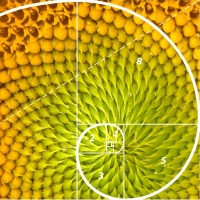In this course, Professor Shabnam Akhtari (University of Oregon) explores proofs, specifically those motivated by number theory and sequences. In the first mini-lecture, we introduce the Fibonacci numbers and use them to motivate examples of...

4 lectures

0:35:43

Prof. Shabnam Akhtari

University of Oregon